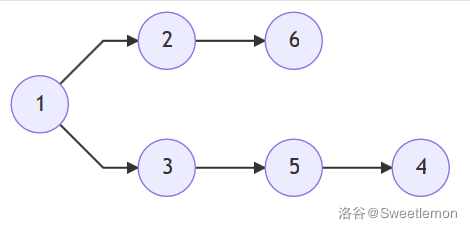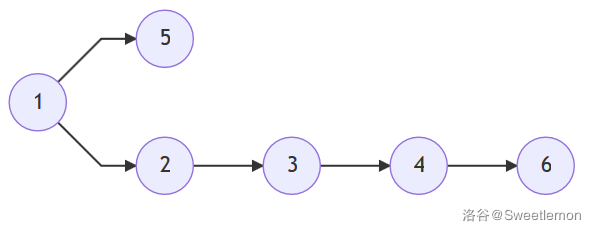# Sweetlemon 的博客# Sweetlemon 的博客

### 贪心？动态规划？都试试！

posted on 2019-10-29 16:48:57 | under 题解 |

#### LGR-062, C, 小 C 与桌游……吗？1. （此时必须买票）选择可访问的点中标号最大的进行访问，代价加 1
2. （此时利用刚买的票）访问可访问点中所有标号小于“最大标号”的点
3. 重复上述两个步骤，直到所有的点都被访问

##### 贪心
#include <cstdio>
#include <cctype>
#include <functional>
#include <algorithm>
#include <queue>
#define MAXIOLG 25
#define MAXN 500005
#define MAXM 1000005
#define INf 114514
#define INF 1919810

using namespace std;

typedef long long ll;
typedef long double ld;
typedef ll io_t;

io_t shin[MAXIOLG];
io_t seto(void); //快读
void ayano(io_t x,char spliter='\n'); //快写

priority_queue<int,vector<int>,greater<int> > q; //小根堆
priority_queue<int,vector<int>,less<int> > q2; //大根堆

int fst[MAXN],nxt[MAXM],edges=0; //存图
int g[MAXM];
int ind[MAXN],visited[MAXN]; //入度, 访问标记

int main(void){
int n,m;
n=seto(),m=seto();
while (m--){
int u,v;
u=seto(),v=seto();
}

int ans1=0,ans2=0;
//先计算尽可能大情况的答案 ans1
for (int i=1;i<=n;i++)
(!ind[i])?(q.push(i)):(void());
int tx=0; //当前访问过的最大的节点
while (!q.empty()){
int nowv=q.top(); //要访问的节点
q.pop();
if (nowv>tx)
tx=nowv,ans1++; //更新答案
for (int ei=fst[nowv];ei;ei=nxt[ei]){
int v=g[ei];
ind[v]--;
(!ind[v])?(q.push(v)):(void());
}
}
ayano(ans1); //输出第一个答案
//再计算尽可能小情况的答案 ans2
for (int i=1;i<=n;i++)
for (int ei=fst[i];ei;ei=nxt[ei])
ind[g[ei]]++; //重新统计入度
for (int i=1;i<=n;i++)
(!ind[i])?(q.push(i),q2.push(i)):(void()),
visited[i]=0; //注意要同时插入到两个堆里
tx=0;
while (!q2.empty()){
//取大根堆堆顶, 买票
int nowv=q2.top();
q2.pop();
if (visited[nowv])
continue;
visited[nowv]=1;
(nowv>tx)?(ans2++,tx=nowv):(0);
//访问这个节点
for (int ei=fst[nowv];ei;ei=nxt[ei]){
int v=g[ei];
ind[v]--;
(!ind[v])?(q.push(v),q2.push(v)):(void());
}
while ((!q.empty()) && (nowv=q.top())<=tx){
//取小根堆堆顶进行访问, 充分利用票
q.pop();
if (visited[nowv])
continue;
visited[nowv]=1;
for (int ei=fst[nowv];ei;ei=nxt[ei]){
int v=g[ei];
ind[v]--;
(!ind[v])?(q.push(v),q2.push(v)):(void());
}
}
}
ayano(ans2); //输出第二个答案
return 0;
}

edges++;
g[edges]=v;
nxt[edges]=fst[u],fst[u]=edges;
}

io_t seto(void){
io_t x=0;
char ch=getchar();
int symbol=0;
while (!isdigit(ch))
(ch=='-')?(symbol=1):(0),ch=getchar();
while (isdigit(ch))
x=(x*10)+(ch-'0'),ch=getchar();
return (symbol)?(-x):(x);
}
void ayano(io_t x,char spliter){
if (!x){
putchar('0'),putchar(spliter);
return;
}
if (x<0)
putchar('-'),x=-x;
int len=0;
while (x){
io_t d=x/10;
shin[len++]=x-d*10;
x=d;
}
while (len--)
putchar(shin[len]+'0');
putchar(spliter);
}
##### 树状数组求区间最值的 dp
#include <cstdio>
#include <cctype>
#include <functional>
#include <algorithm>
#include <queue>
#define MAXIOLG 25
#define LOWBIT(x) ((x)&(-(x)))
#define MAXN 500005
#define MAXM 1000005
#define INf 114514
#define INF 1919810
using namespace std;

typedef long long ll;
typedef long double ld;
typedef ll io_t;

io_t shin[MAXIOLG];
io_t seto(void);
void ayano(io_t x,char spliter='\n');

priority_queue<int,vector<int>,greater<int> > q;

int n;
int fst[MAXN],nxt[MAXM],edges=0;
int g[MAXM];
int ind[MAXN];
int pre[MAXN]; //上文所述的 pre 数组
int f[MAXN]; //上文所述的动态规划 f 数组
int ftarr[MAXN]; //用于存储 f 最小值的树状数组

int query(int l,int r); //树状数组查询区间最小值, O(log n log n)
void update(int x,int v); //简化版的树状数组更新区间最小值

int main(void){
int m;
n=seto(),m=seto();
while (m--){
int u,v;
u=seto(),v=seto();
}
for (int i=1;i<=n;i++)
(!ind[i])?(q.push(i),pre[i]=0):(0),
f[i]=ftarr[i]=INF; //初始化, f 和树状数组都初始化为 INF
int ans1=0; //第一行输出的答案
int tx=0; //当前访问的最大节点(在计算第一个答案时用)
//由于 dp 可以按照任意拓扑序计算
//因此本程序中两个答案的计算同时进行
while (!q.empty()){
int nowv=q.top();
q.pop();
//下面一部分用于 dp
if (nowv>pre[nowv]){
//关键点
f[nowv]=query(pre[nowv],nowv-1)+1; //查询区间最值, 加1
update(nowv,f[nowv]); //插入到树状数组中进行更新
}
int tpre=max(pre[nowv],nowv);
//下面一部分用于贪心
if (nowv>tx)
tx=nowv,ans1++;
for (int ei=fst[nowv];ei;ei=nxt[ei]){
int v=g[ei];
pre[v]=max(pre[v],tpre); //在访问的同时更新 pre
ind[v]--;
(!ind[v])?(q.push(v)):(void());
}
}
//输出两个答案
ayano(ans1);
ayano(f[n]);
return 0;
}

int query(int l,int r){
//查询区间 [l,r] 的最小值
if (!l)
return 0; //注意树状数组遇到下标 0 会出事, 所以提前处理; f=0
int ans=INF;
while (r>=l){
int t=r^LOWBIT(r); //相当于 t=r-LOWBIT(r)
if (t>=l)
ans=min(ans,ftarr[r]),r=t;
else
ans=min(ans,f[r]),r--;
}
return ans;
}

void update(int x,int v){
//注意,这是简化版的更新函数
//由于本题中树状数组中的值只会变小不会变大
//所以可以像求和一样进行更新, 更新复杂度 O(log n)
//但是一般的 值有可能变大的问题不能这么写
//必须对所有有影响的点重新计算最值, 更新复杂度 O(log n log n)
while (x<=n)
ftarr[x]=min(ftarr[x],v),
x+=LOWBIT(x);
}
//以下略去了 addedge, seto, ayano 三个函数的实现

#### set 优化 dp

#include <cstdio>
#include <cctype>
#include <functional>
#include <algorithm>
#include <queue>
#include <map>
#include <set>
#define MAXIOLG 25
#define MAXN 500005
#define MAXM 1000005
#define INf 114514
#define INF 1919810

using namespace std;

typedef long long ll;
typedef long double ld;
typedef ll io_t;

io_t shin[MAXIOLG];
io_t seto(void);
void ayano(io_t x,char spliter='\n');

priority_queue<int,vector<int>,greater<int> > q; //用于贪心的小根堆
set<pair<int,int> > st; //pair 自带比较函数

int fst[MAXN],nxt[MAXM],edges=0;
int g[MAXM];
int ind[MAXN];
int pre[MAXN];
int f[MAXN];

int main(void){
int n,m;
n=seto(),m=seto();
while (m--){
int u,v;
u=seto(),v=seto();
}
for (int i=1;i<=n;i++)
(!ind[i])?(q.push(i),pre[i]=0):(0),
f[i]=INF;
int ans1=0;
int tx=0;
f=0;
st.insert(make_pair(0,0));
while (!q.empty()){
int nowv=q.top();
q.pop();
int tpre=pre[nowv];
//以下是 dp
if (nowv>tpre){
//计算 f 值; first 保存"值"——标号,second 保存"下标"——代价
f[nowv]=st.lower_bound(make_pair(tpre,0))->second+1;
int tf=f[nowv];
//下面将把 f[nowv] 插入到 set 中
//找到标号小于 nowv 且代价大于 nowv 的状态
auto it=st.lower_bound(make_pair(nowv,0));
// 不断删除
while ((it!=st.begin() && (it--,1)) && (it->second > tf))
st.erase(it),it=st.lower_bound(make_pair(nowv,0));
//插入
st.insert(make_pair(nowv,tf));
}
tpre=max(tpre,nowv);
//以下是贪心
if (nowv>tx)
tx=nowv,ans1++;
for (int ei=fst[nowv];ei;ei=nxt[ei]){
int v=g[ei];
pre[v]=max(pre[v],tpre);
ind[v]--;
(!ind[v])?(q.push(v)):(void());
}
}
ayano(ans1);
ayano(f[n]);
return 0;
}
//以下略去了 addedge, seto, ayano 三个函数的实现